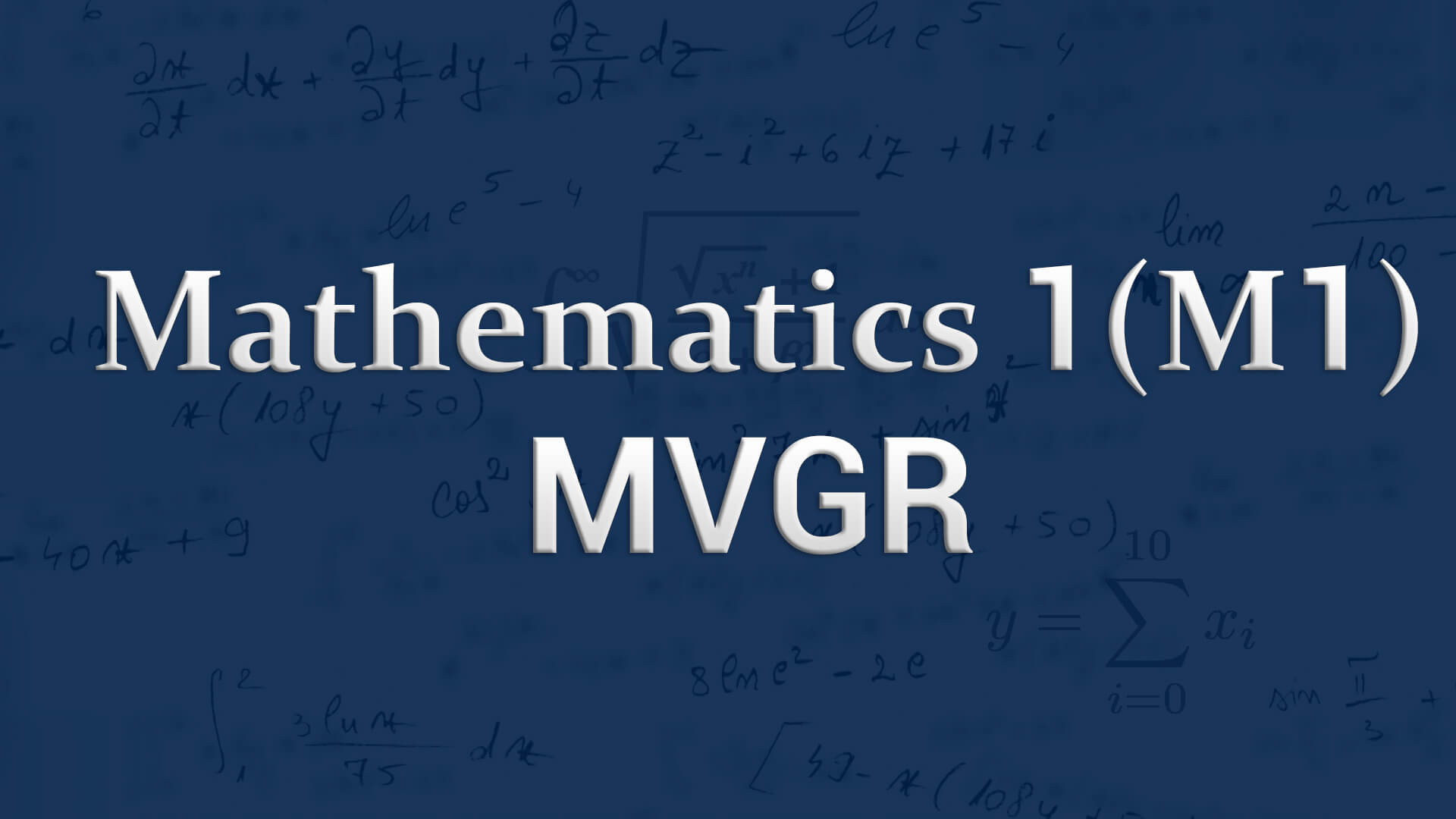## Mathematics 1 for MVGR by Mr C Srinivas MSc ,BEd (Osmania) The course is designed to equip the students with the necessary mathematical skills and techniques that are essential for an engineering course. The skills derived from the course will help the student from a necessary base to develop analytic and design concepts. Code : M1MVGRB.tech1 Recommended For : B.Tech 1st Year

#### After Learning this subject,you should be able to

• Solve linear differential equations of first, second and higher order.
• Determine Laplace transform and inverse Laplace transform of various functions and use Laplace transforms to determine general solution to linear ODE.
• Calculate total derivative, Jocobian and minima of functions of two variables.

## Curriculum 11:23:54   units   |   8

• 1:29:12

UNIT 1
Differential equations of first order: Exact differential equations, Reducible to exact Part 1, Reducible to exact Part 2, Linear and Bernoulli Equations, Applications of differential equations of first order, Newtons law of cooling and natural growth and decay, Problems on Newtons law of cooling, Problems on natural growth and decay, Orthogonal trajectories, Problems on Orthogonal Trajectories Part 1, Problems on Orthogonal Trajectories Part 2

• 1:41:25

UNIT 2
Higher order linear differential equations: Linear Differential Equations & Rules for Finding Complimentary Function, Linear Differential Equations & Rules for Finding Complimentary Function 1, operator form, Rules for Finding Particular Integral, Rules for Finding Particular Integral 1, working procedure to solve the equations, Higher order differentional equations Problem 1, Higher order differentional equations Problem 2, Higher order differentional equations Problem 3, Higher order differentional equations Problem 4, Higher order differentional equations Problem 5, Higher order differentional equations Problem 6, Higher order differentional equations Problem 7, Higher order differentional equations Problem 8, Higher order differentional equations Problem 9, Higher order differentional equations Problem 10

• 2:10:26

UNIT 3
Laplace Transforms: Introduction, definition, transforms of elementary functions, transforms of elementary functions-PART 01, transforms of elementary functions Problems, transforms of elementary functions Problems Part 1, First Shifting theorem, Problems on First Shifting theorem, Properties of laplace transforms, properties of laplace transforms Part 1, Transform of Derivatives & Inegrals, Problems transforms of derivatives, transforms of integrals, Multiplication by t power n& Division by t, multiplication by t power n, division by t (statements only)

• 1:25:48

UNIT 3.1
Inverse transforms: Introduction, Basic Formulas of Inverse Laplace Transform, Problems on Inverse Laplace Transform, Method of Partial fraction, Method of Partial fraction Part 1, First Shifting theorem, Problem on First Shifting theorem, Second Shifting Theorem, Inverse Laplace Transform of Derivatives&Integrals, Multiplication & Division by S, Convolution theorem, Convolution theorem (without proof), applications to differential equations, applications to differential equations - Part 1

• 0:50:41

UNIT 4
Partial differentiation and its applications: Functions of two or more variables, total derivative, differentiation of implicit function, change of variables, Jacobians, Jacobian Problem1, Jacobian Problem2, Lagranges Method, Max and min of functions of 2 & 3 variables using method of Lagrange Multipliers Part 1, Max and min of functions of 2 & 3 variables using method of Lagrange Multipliers Part 2, Max and min of functions of 2 & 3 variables using method of Lagrange Multipliers Part 3

• 2:05:26

UNIT 5
Partial differential equations: Formation of partial differential equations by elimination of arbitrary constants, Formation of partial differential equations by elimination of arbitrary functions, Formation Of P.D.E By Eliminating arbitrary Constants - Problem 1, Formation Of P.D.E By Eliminating arbitrary Constants - Problem 2, Formation Of P.D.E By Eliminating arbitrary Constants - Problem 3, Formation of PDE By arbitrary functions - Problem 1, Formation of PDE By arbitrary functions - Problem 2, Formation of PDE By arbitrary functions - Problem 3, Formation of PDE By arbitrary functions - Problem 4, Lagranges method to solve the first order linear equations, Lagranges Linear Equation Part 1, Lagranges Linear Equation Part 2, Solutions Of Non Linear Of PDA, The standard type methods to solve the non linear equations Part 1, The standard type methods to solve the non linear equations Part 2, The standard type methods to solve the non linear equations Part 3, Standard Form II Problem, Standard Form II Problem 1, Standard Form II Problem 2, Standard Form III & IV, Standard Form V &VI - Problem 1, Standard Form V &VI - Problem 2

• 0:51:23

UNIT 6
Higher order Partial differential equations: Homogeneous linear partial differential equations with constant coefficients, Homogeneous linear partial differential equations with constant coefficients Part 1, Homogeneous linear partial differential equations with constant coefficients Part 2, Rules for finding complimentary function, rules for finding particular integral, working procedure to solve the equations

• 0:49:33

UNIT 6.1
Applications of partial differential equations: Method of separation of variables, Problems on Method of separation of variables, vibrations of stretched string, wave equation, Problems on Wave Equation, One-dimensional heat flow equation, solution of Laplace equation (problems only)